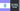# How to Convert string to integer type in Go?

07 Apr, 2022, 3 min read

# How to Convert string to integer type in Go?

You can convert string to integer type in golang using the `Atoi()` function from the `strconv` package. It is useful to convert any numbers which are in string format to an integer type to perform mathematical operations.

There are two main methods to use to convert string to integer

1. `Atoi()` function from `strconv` package for the decimal string for simple conversion.
2. `ParseInt()` function from `strconv` package for more advanced parameters. It can be used to convert binary/decimal representations of strings to integers.

## Convert string to integer using Atoi() - Method 1

``````package main

import (
"fmt"
"strconv"
)

func main() {
marksStr := "320"
marks, err := strconv.Atoi(marksStr)

if err != nil {
fmt.Println("Error during conversion")
return
}

fmt.Println(marks)
}``````

Output

``320``

## Syntax of the Atoi() function

``func Atoi(s string) (int, error)``

Atoi() function represents the conversion of ASCII value to an integer value and it is part of the `strconv` package. The function takes a string as an argument and returns an integer value and error.

Check if the error is not nil and then you can use the integer value which will be in `int` type

Atoi() function returns the value of `ParseInt()` function with a base of `10` and bit value of `0`. You can learn more about the `ParseInt()` in the next section

# Convert string to int using ParseInt() - Method 2

ParseInt() function takes three arguments and returns an integer and error value. The string to convert to integer is the first argument, the base of the conversion is the second argument and the bit value is the third argument.

``````package main

import (
"fmt"
"strconv"
)

func main() {
marksStr := "320"
marks, err := strconv.ParseInt(marksStr, 10, 0)

if err != nil {
fmt.Println("Error during conversion")
return
}

fmt.Println(marks)
}``````

Output

``320``

## Syntax of ParseInt() function

``func ParseInt(s string, base int, bitSize int) (i int64, err error)``

The first argument is the string which will be used to try and parse it to the integer based on the other two arguments. The second argument is the base which is the numeric base to convert. When converting to a decimal system (digits from 0 to 9), you can use the base of `10`. Base 10 will be most used since most of the applications will be working on a Decimal numerical system

## Conversion of binary string to integer

If you want to convert the integer which is in binary format, you can use the base of `2`

``````package main

import (
"fmt"
"strconv"
)

func main() {
marksStr := "1010"
marks, err := strconv.ParseInt(marksStr, 2, 0)

if err != nil {
fmt.Println("Error during conversion")
return
}

fmt.Println(marks)
}``````

Output

``10``

You can give the binary number which is in string format as the first argument. The second argument should be `2` which means the string is represented in binary format.

## Conversion of string to 32-bit integer

You can use the third argument of the `ParseInt` function to specify the number of bits (0 to 64)

``````package main

import (
"fmt"
"reflect"
"strconv"
)

func main() {
marksStr := "320"
marks, err := strconv.ParseInt(marksStr, 10, 32)

fmt.Println(marks, err, reflect.TypeOf(marks))

outOfBound := "250"
outInt, err2 := strconv.ParseInt(outOfBound, 10, 5)

fmt.Println(outInt, err2, reflect.TypeOf(outInt))
}``````

Output

``````320 <nil> int64
15 strconv.ParseInt: parsing "250": value out of range int64``````

If you provide a string that is out of the range of the bits, you will error return from the function. Since “250” cannot be represented in `5` bits, you are getting an error.

Golang Maps

Golang Switch